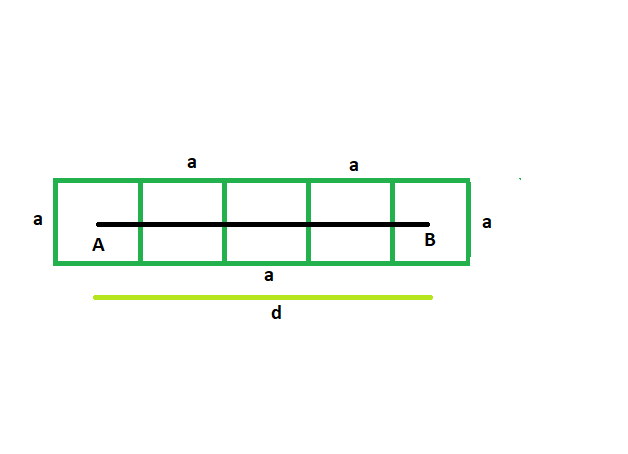# Find the side of the squares which are lined in a row, and distance between the centers of first and last square is given

Given here are n squares which touch each other externally, and are lined up in a row. The distance between the centers of the first and last square is given. The squares have equal side length. The task is to find the side of each square.

Examples:

```Input: d = 42, n = 4
Output: The side of each square is 14

Input: d = 36, n = 5
Output: The side of each square is 9
```Approach:
Suppose there are n squares each having side of length a.
Let, the distance between the first and last squares = d
From the figure, it is clear,
a/2 + a/2 + (n-2)*a = d
a + na – 2a = d
na – a = d

so, a = d/(n-1)## C++

 `// C++ program to find side of the squares ` `// which are lined in a row and distance between the ` `// centers of first and last squares is given ` ` `  `#include ` `using` `namespace` `std; ` ` `  `void` `radius(``int` `n, ``int` `d) ` `{ ` `    ``cout << ``"The side of each square is "` `         ``<< d / (n - 1) << endl; ` `} ` ` `  `// Driver code ` `int` `main() ` `{ ` `    ``int` `d = 42, n = 4; ` `    ``radius(n, d); ` `    ``return` `0; ` `} `

## Java

 `// Java program to find side of the squares ` `// which are lined in a row and distance between the ` `// centers of first and last squares is given ` `import` `java.io.*; ` ` `  `class` `GFG ` `{ ` ` `  `static` `void` `radius(``int` `n, ``int` `d) ` `{ ` `    ``System.out.print( ``"The side of each square is "` `        ``+ d / (n - ``1``)); ` `} ` ` `  `// Driver code ` `public` `static` `void` `main (String[] args)  ` `{ ` `    ``int` `d = ``42``, n = ``4``; ` `    ``radius(n, d); ` `} ` `} ` ` `  `// This code is contributed by vt_m. `

## Python3

 `     `  `# Python program to find side of the squares ` `# which are lined in a row and distance between the ` `# centers of first and last squares is given ` ` `  `def` `radius(n, d): ` ` `  `    ``print``(``"The side of each square is "``, ` `          ``d ``/` `(n ``-` `1``)); ` ` `  ` `  `d ``=` `42``; n ``=` `4``; ` `radius(n, d); ` ` `  ` `  ` `  `# This code contributed by PrinciRaj1992 `

## C#

 `// C# program to find side of the squares ` `// which are lined in a row and distance between the ` `// centers of first and last squares is given ` `using` `System; ` ` `  `class` `GFG ` `{ ` ` `  `static` `void` `radius(``int` `n, ``int` `d) ` `{ ` `    ``Console.Write( ``"The side of each square is "` `        ``+ d / (n - 1)); ` `} ` ` `  `// Driver code ` `public` `static` `void` `Main ()  ` `{ ` `    ``int` `d = 42, n = 4; ` `    ``radius(n, d); ` `} ` `} ` ` `  `// This code is contributed by anuj_67.. `

Output:

```The side of each square is 14
```

Attention reader! Don’t stop learning now. Get hold of all the important DSA concepts with the DSA Self Paced Course at a student-friendly price and become industry ready.

My Personal Notes arrow_drop_upCheck out this Author's contributed articles.

If you like GeeksforGeeks and would like to contribute, you can also write an article using contribute.geeksforgeeks.org or mail your article to contribute@geeksforgeeks.org. See your article appearing on the GeeksforGeeks main page and help other Geeks.

Please Improve this article if you find anything incorrect by clicking on the "Improve Article" button below.

Improved By : vt_m, princiraj1992

Article Tags :
Practice Tags :

Be the First to upvote.

Please write to us at contribute@geeksforgeeks.org to report any issue with the above content.